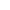﻿ 3 digit subtraction with / without regrouping games online

Page No. 1005

# 3 digit subtraction games

Play 3 digit subtraction games online, choose between two options: 3 digit subtraction with regrouping + 3 digit subtraction without regrouping online games.

------------------------------------------------------------

New gameFill in the result of 3 digit subtraction to the empty boxes.
Choose ´Without regrouping´ or ´With regrouping´.

## 3 digit subtraction games onlineInsert the result of 3 digit subtraction game to the empty boxes. Each box should contain only 1 digit. Press "Check it" to find out if the result is correct. If your outcome is OK, the box will be green. If your answer isn´t correct, the box will be red and you will see the correct digit.

Press the button "New game" and play another three digit subtraction game.

## Free printable 3 digit subtraction worksheets PDF

I have included these 3 digit subtraction worksheets in PDF with answer keys. You can generate free printable three digit subtraction with regrouping worksheets or 3 digit subtraction without regrouping worksheets. You can find more subtraction worksheets here.

## 3 digit subtraction online games

It's best to start with 3 digit subtraction without regrouping games and once the kids learn it, they can continue to solve 3 digit subtraction with regrouping games.

• ### 3 digit subtraction without regrouping games online

Click on the blue button No regrouping and play 3 digit subtraction without regrouping online games.

• ### 3 digit subtraction with regrouping games online

Click on the blue button Regrouping and you start to play 3 digit subtraction with regrouping online games. Sometimes you have to regroup in one column, in some cases you will regroup in two or all three columns.

These 3 digit subtraction online games teach kids 3 digit subtraction with and without regrouping. You can play three digit subtraction games online on any device. No download needed, only internet connection.

For better understanding of subtraction 3 digit numbers watch this tutorial on YouTube.

## 3 digit subtraction: regrouping games online

In this 3 digit subtraction game we use a column subtraction method for regrouping, which is the best way to make big calculations easy.

If the children have mastered 2 digit subtraction, this three digit subtraction will be a piece of cake for them.

• ### Three digit subtraction without borrowing

When you subtract without regrouping (borrowing), all of the numbers you're subtracting are smaller than the digits you're subtracting from.

In the column subtraction method it means than the upper digit is larger or equal than the digit below it.

• ### Three digit subtraction with borrowing

Three digit subtraction with borrowing is used (in the column method) when the minuend (the number from which another is to be subtracted) is smaller than the subtrahend (the number to be subtracted from minued).

## How to do three digit subtraction

For example, let’s take the problem: 747 - 489. Starting with the units (the right column), you can’t take 9 away from 7.

So, we have to borrow 1 from the tens column. We are now subtracting 9 from 17. It is 8.

We continue to subtract the tens column, the middle column in this case. Since, 1 ten is regrouped by ones column, so we will subtract 8 from 3 (4-1). It is not possible, so we borrow 1 from hundreds and will subtract 8 from 13. It is 5.

Finally, we subtract the hundreds column, the left one. We subtract 4 from 6 (7-1 because we borrowed 1 in the previous step). The result is: 6 - 4 = 2.

747
- 489
--------
258

## Subtraction 3 digit numbers

3 digit numbers are numbers that consist of 3 digits. They begin with 100 and end on 999. In our 3 digit subtraction game numbers are randomly generated in this range.

We use regrouping in subtraction problems when digits in the minuend are smaller than the digits in the same place in the subtrahend.

When subtracting three digit numbers 3 digit numbers we have to understand changing pattern in each of the digits of the hundredth place, ten's place, and unit's place, as we explained in the example above.